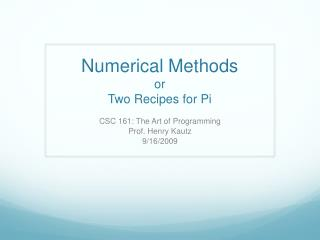DownloadDownload PresentationNumerical Methods or Two Recipes for Pi

# Numerical Methods or Two Recipes for Pi

Télécharger la présentation## Numerical Methods or Two Recipes for Pi

- - - - - - - - - - - - - - - - - - - - - - - - - - - E N D - - - - - - - - - - - - - - - - - - - - - - - - - - -
##### Presentation Transcript

1. Numerical MethodsorTwo Recipes for Pi CSC 161: The Art of Programming Prof. Henry Kautz 9/16/2009

2. A Fable • The Maharaja of India was so pleased with one of his palace wise men, who had invented the game of chess, that he offered him a reward of his own choosing. • The wise man told his Master that he would like just one grain of rice on the first square of the chess board, double that number of grains of rice on the second square, and so on: double the number of grains of rice on each of the next 62 squares on the chess board. • This seemed to the ruler to be amodest request, so he called forhis servants to bring the rice.

3. Rice def rice(): print "Square", "Grains" amount = 1 for sin range(1,64): print s, amount amount = amount * 2 1 is starting value

4. Minute Rice • How can we compute the amount of rice on the last square in one line? • 2 ** 63

5. Compound Interest def compound_interest(principle, \yearly_rate, months): print "Month", "Principle" monthly_rate = yearly_rate / 12.0 for min range(months): print m, principle principle = principle + \monthly_rate* principle

6. Compound Interest with Fixed Payment def compound_interest(principle, \ yearly_rate, months, monthly_payment): print "Month", "Principle" monthly_rate = yearly_rate / 12.0 for min range(months): print m, principle principle = principle + \monthly_rate* principle – \monthly_payment

7. Compound Interest Until Paid Off def compound_interest(principle, yearly_rate, \monthly_payment): print "Month", "Principle" monthly_rate = yearly_rate / 12.0 m = 0 while principle >= 0: print m, principle principle = principle + monthly_rate* \ principle - monthly_payment m = m + 1

8. Two Recipes for Pi

9. Recipe the First: the Gregory-Leibniz Series What ingredients do we need to make Pi?

10. Gergory-Leibniz Pi from 1 to terms-1 by 4 def make_pi(terms): print "Terms", "Estimate" p = 0 for din range(1,terms,4): p = p + (4.0/d) - (4.0/(d+2)) print d, p

11. Recipe the Second: Dartboard Pi

12. Dartboard Pi • Suppose circular dartboard fit exactly into a 1 foot square • Suppose you throw darts that are equally likely to hit any spot in the square • The proportion of darts that hit the circle is proportional to the area of the circle

13. Dartboard Pi • The proportion of darts that hit the circle is proportional to the area of the circle

14. Dartboard Pi import math import random def make_pi(darts): hits = 1 for iin range(darts): dx = random.random() dy = random.random() if math.sqrt((0.5-dx)**2 + \ (0.5-dy)**2) < 0.5: hits = hits + 1 print "I think Pi is", (4.0*hits)/darts

15. Monte-Carlo Algorithms • This is an example of what is called a Monte-Carlo Algorithm • One that uses random samples to estimate some value • These kinds of algorithms are used throughout • Chemistry • Biology • Physics • Economics • Wherever you need to estimate some quantity that is hard to determine exactly!

16. Course Status • Assignment 2: Turn in by 10:00am Saturday • Pre-read: Zelle, Chapter 4 • Workshop: Mystery Topic